title: “Introduction-to-doremi” author: “Adriana Uribe” date: “2018-08-22” output: rmarkdown::html_vignette vignette: > % % %

The main purpose of the Dynamics Of Return to Equilibrium during Multiple Inputs (doremi) model included in this package is to be able to fit trends of homeostasis and return to equilibrium in noisy data. Doremi estimates coefficients for the solution of a first order differential equation using linear mixed-effects (multilevel) models. The differential equation considered is the following:

$\frac{1}{\gamma} \dot{y}(t) + y(t) = \epsilon E(t) + eqvalue (1)$

Where the variables:

• $$y(t)$$ is the signal to be analyzed

• $$\dot{y}(t)$$ is its first derivative

• $$E(t)$$ is the excitation term creating the dynamics of $$y(t)$$

And regarding the coefficients:

• $$\gamma$$ is the damping rate, the inverse of which is the damping time. The damping rate indicates the relative variation of the signal with respect to time when the excitation term is 0. The damping time is the characteristic response time of the solution to equation (1). When the excitation starts, the signal reaches its new value and $$\tau =\frac{1}{\gamma}$$ corresponds to the time needed to reach 63% of the difference between the maximum value and the initial value. After an excitation, when the signal returns to a lower equilibrium level, the damping time corresponds to the time needed to reach ~37% (1/e) of the difference between the level reached and the equilibrium value. Figure 1 presents both the damping time $$\tau$$ for the increase and decrease of the signal. Further development of the model will relax this constraint and will allow for different signal increase and decrease behavior.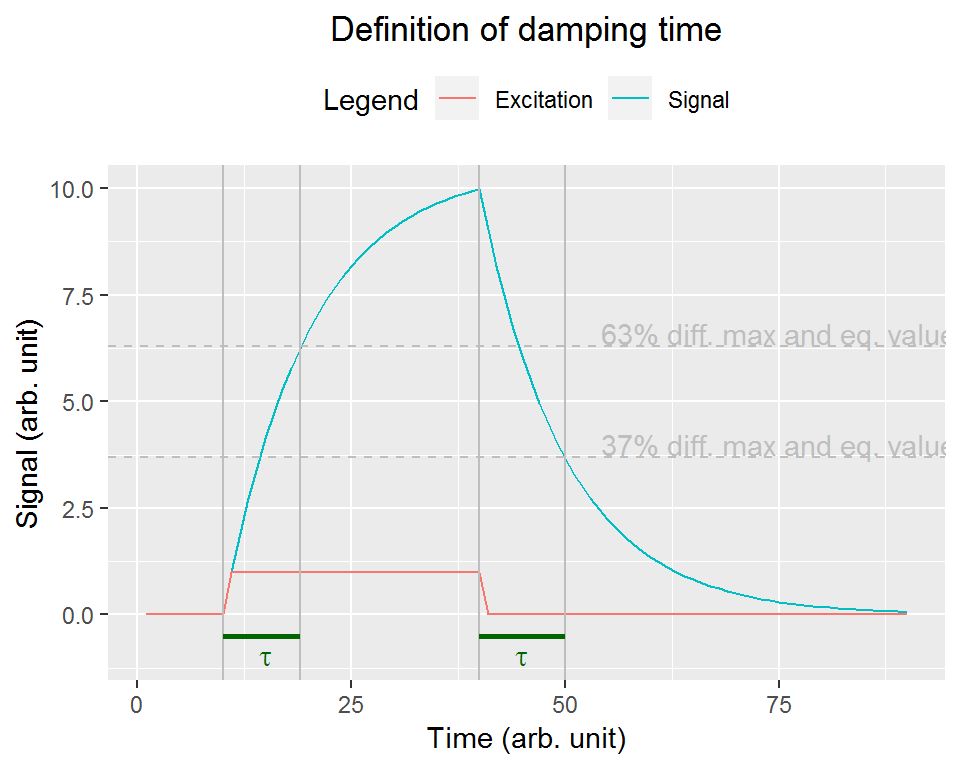• $$\epsilon$$ is the excitation coefficient, also called “gain” in control theory. It indicates the proportionality between the maximal amplitude the signal can reach and the input (also called excitation).

• eqvalue is the equilibrium value, that is, the value the system has when there is no excitation and no remaining effect of previous excitation (i.e., when t tends to infinity).

In order to find these coefficients, the model performs the following linear mixed-effect regression derived from equation (1), as presented by [(Mongin et al., under review)]:

$\dot{y}_{ij} \sim b_{0}+b_{0j}+b_{1}y_{ij}+b_{2}E_{ij}+u_{1j}y_{ij}+u_{2j}E_{ij}+e_{ij} (2)$

where:

• i accounts for the time

• j accounts for the different individuals

• $$\dot{y}_{ij}$$ is the derivative calculated on embedding points through the GOLD method (Deboeck, 2010) to avoid the appearance of correlated errors in the estimation

• $$y_{ij}$$ and $$E_{ij}$$ are the signal and the excitation averaged on embedding points

• $$e_{ij}$$ is the error term

Note that random effects are estimated for the intercept ($$b_{0} +b_{0j}$$), signal ($$b_{1} +u_{1j}$$) and excitation terms ($$b_{2} +u_{2j}$$), so that individuals can vary on their initial level, their evolution over time and their reaction to an excitation.

The coefficients aforementioned can be thus calculated as:

• Damping time: $$\tau_{j} = \frac{1}{\gamma_{j}}$$ with $$\gamma_{j} = b_{1} + u_{1j}$$

• Excitation coefficient: $$\epsilon_{j} = \frac{b_{2} + u_{2j}}{\gamma_{j}}$$

• Equilibrium value: $$eqvalue_{j} = \frac{b_{0} + b_{0j} }{\gamma _{j}}$$

The estimation is performed using the function lmer if there are several individuals or lm if there is only one.

With the above estimated parameters, the estimated signal can be reconstructed for each individual as the analytical solution to equation (1) is known:

$y(t) = \frac{\epsilon}{\tau}\int E(t')G(t-t')dt'+ eqvalue (3)$

Where y(0) = eqvalue and G(t) is the Green function, that is, the function that satisfies the differential equation when the excitation term is a Dirac delta function. In our case, it is a decreasing exponential of the form: $G(t) = exp(\frac{-t}{\tau}) (4)$

The estimated signal is then built by first performing the convolution of the excitation with the Green function -using the estimated damping rate- and then offsetting the resulting signal with the equilibrium value.

The doremi package contains three types of functions:

### Simulation functions

These are functions that allow the user to create data corresponding to a first order differential equation (i.e. solution of equation (1)). The simulation functions presented in the file are:

• generate.remi: creates the solution of equation (1) for a given damping time and excitation vector.

• generate.panel.remi: creates a set of signals that are solution of equation (1) for random simulated excitations. Number of points, damping time, intra-individual noise and inter-individual noise can be controlled.

### Analysis functions

The analysis function presented in the package is:

• remi: the function performs the presented mixed-effects regression (2) and provides the calculated derivatives, the differential equation coefficients per individual and averaged, the fixed and random coefficients coming from the fit and the estimated signals, according to (3).

### Auxiliary functions

These functions are used by the simulation and/or analysis functions but they can also be used independently:

• generate.excitation: generates a random succession of squared pulses for a given number of points, number of pulses, amplitude and duration (used for simulation purposes only).

• calculate.gold: calculates the derivative of a group of data points by using the GOLD method (Deboeck, 2010) extended to treat non-constant time steps (used in the analysis function only).

# A - Simulating data

In this section, we present increasingly complex examples of data simulation.

## Example 1 - Generating signals with no noise

Generating data for 4 individuals, with a damping time of 10s for an excitation vector formed by 3 pulses of amplitude 1 and duration 10s distributed randomly in a time period of 100s, with a minimum spacing between pulses of 20 s and with no noise. That is, the signal follows exactly the differential equation (no intraindividual noise) with no variation of the damping time, the excitation coefficient and the equilibrium value across individuals (no interindividual noise). The amplitude of the generated signal is set so that $$\tau * \gamma = 1$$ in equation (3).

mydataa1 <- generate.panel.remi(nind = 4,
dampingtime = 10,
amplitude = 1,
nexc = 3,
duration = 10,
deltatf = 0.5,
tmax = 100,
minspacing = 20,
internoise = 0,
intranoise = 0)

The function returns an object of the class ‘doremidata’ that contains two data.frame that can be visualized using the str or head functions. Entering the variable name directly will display the first and last lines of the $data data.frame, that contains the data sampled at deltatf intervals. mydataa1 #>  "$data"
#>      id excitation  time dampedsignalraw dampedsignal
#>   1:  1          0   0.0    4.418341e-13 4.418341e-13
#>   2:  1          0   0.5    4.233999e-13 4.233999e-13
#>   3:  1          0   1.0    4.058395e-13 4.058395e-13
#>   4:  1          0   1.5    3.905430e-13 3.905430e-13
#>   5:  1          0   2.0    3.767533e-13 3.767533e-13
#>  ---
#> 800:  4          0  98.0    8.051926e-01 8.051926e-01
#> 801:  4          0  98.5    7.659229e-01 7.659229e-01
#> 802:  4          0  99.0    7.285684e-01 7.285684e-01
#> 803:  4          0  99.5    6.930357e-01 6.930357e-01
#> 804:  4          0 100.0    6.592360e-01 6.592360e-01

Where:

• id is the identifier of the individual

• excitation is the excitation signal

• time is the time column generated.

• dampedsignalraw is the signal without noise

• dampedsignal is the signal with noise (in this case, the values of this column are the same as those of the dampedsignalraw column and in the plot, you will see that both lines are overlapped).

Plotting the data:

ggplot2::ggplot( data = mydataa1$data ) + ggplot2::geom_line(ggplot2::aes(time,dampedsignalraw, colour = "dampedsignalraw"))+ ggplot2::geom_point(ggplot2::aes(time,dampedsignal, colour = "dampedsignal"))+ ggplot2::geom_line(ggplot2::aes(time,excitation,colour = "Excitation"))+ ggplot2::facet_wrap(~id)+ ggplot2::labs(x = "Time (s)", y = "Signal (arb. unit)", colour = "")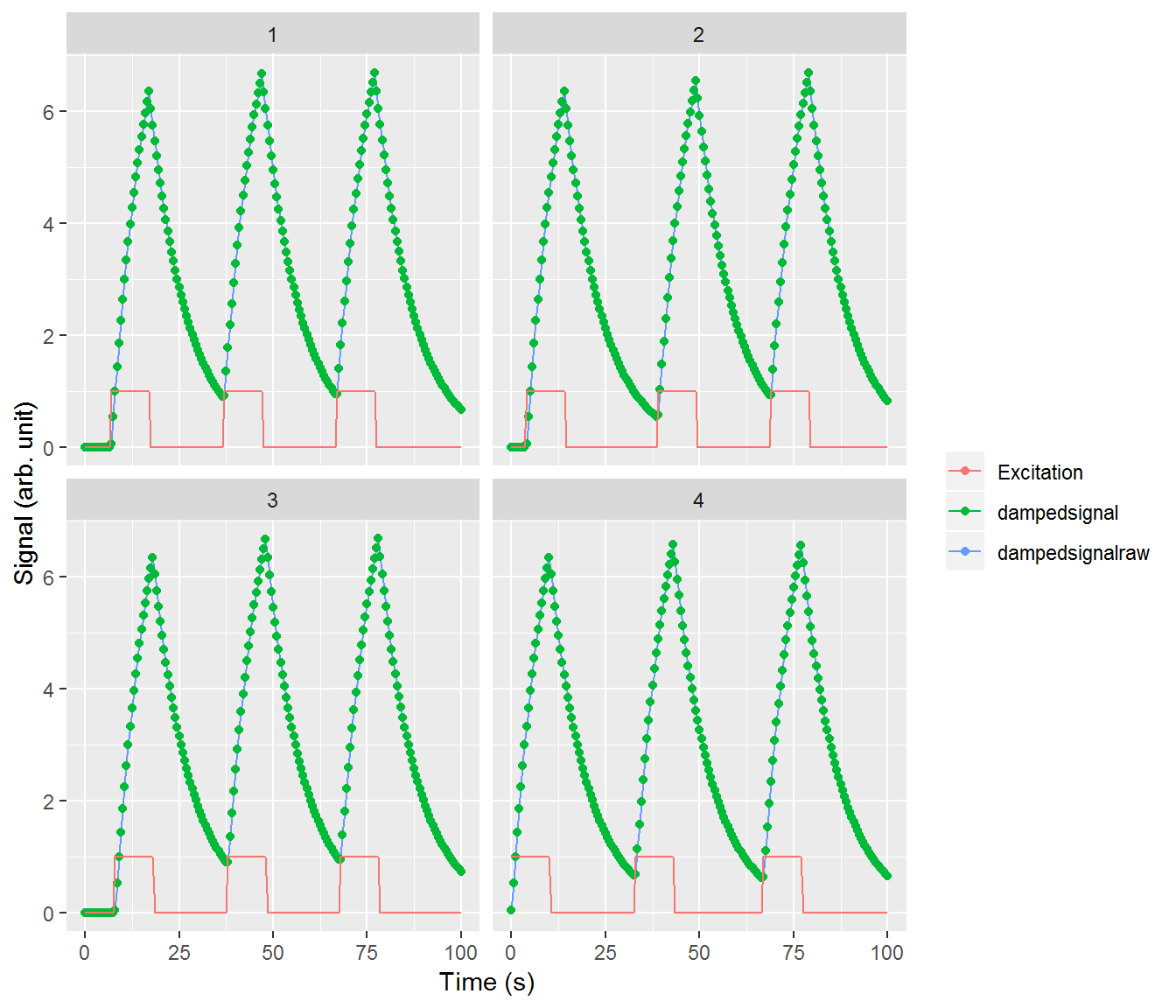## Example 2 - Generating signals with noise The call to the function remains almost the same, this time adding a 20% intra-individual noise and a 40% inter-individual noise: # Generation of signals with intra and inter-noise mydataa2 <- generate.panel.remi(nind = 4, dampingtime = 10, amplitude = 1, nexc = 3, duration = 10, deltatf = 0.5, tmax = 100, minspacing = 20, internoise = 0.4, intranoise = 0.2) # Plotting the data ggplot2::ggplot( data = mydataa2$data ) +
ggplot2::geom_line(ggplot2::aes(time,dampedsignalraw, colour = "Signal-no noise"))+
ggplot2::geom_point(ggplot2::aes(time,dampedsignal, colour = "Signal with 20% intra-noise"))+
ggplot2::geom_line(ggplot2::aes(time,excitation,colour = "Excitation"))+
ggplot2::facet_wrap(~id)+
ggplot2::labs(x = "Time (s)",
y = "Signal (arb. unit)",
colour = "")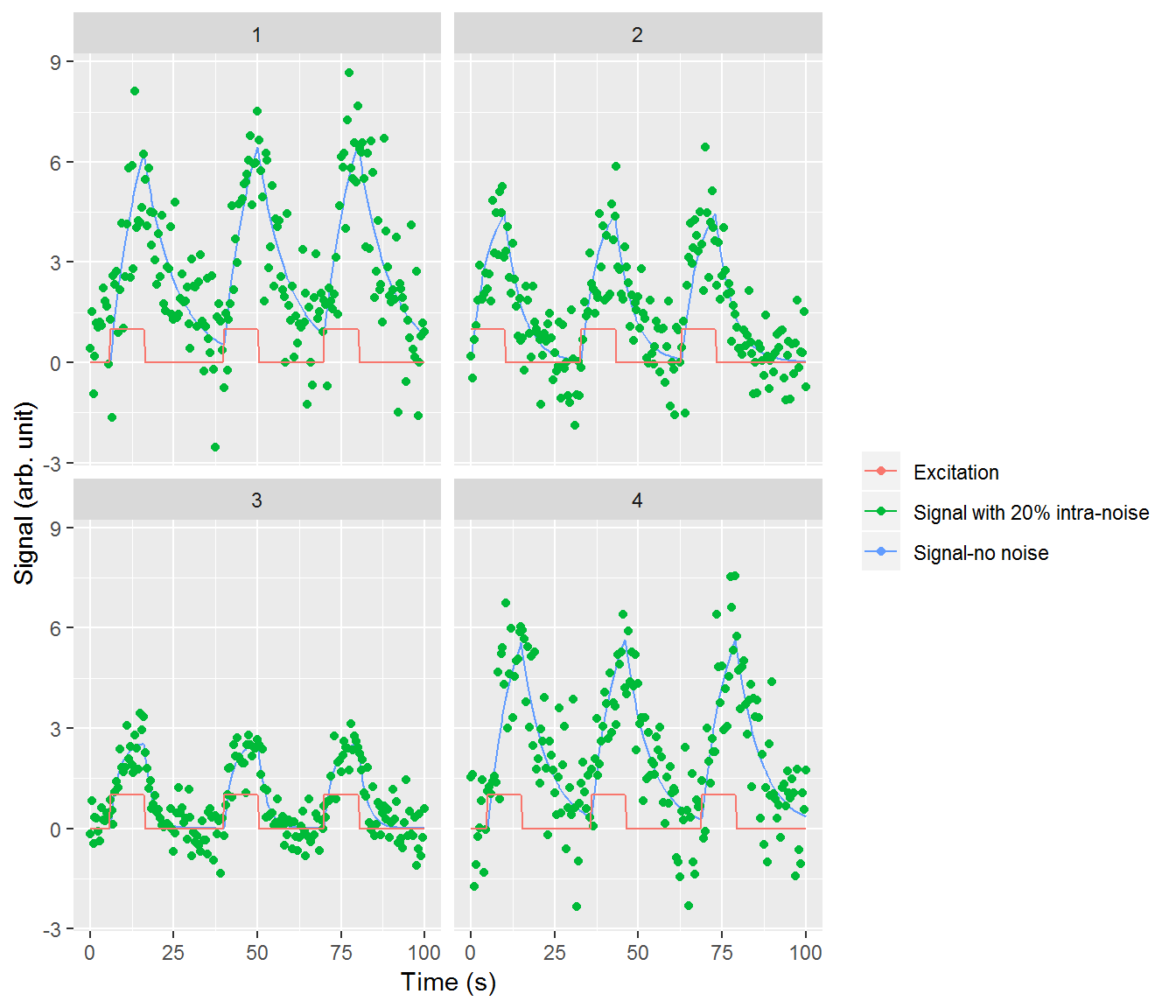# B - Analyzing data

Analyzing the signals with noise generated above, we can verify that the damping coefficient was the one introduced in the simulation function and that the estimated signals generated match the simulated one.

## Example 1 - Analyzing data with several individuals and some inter and intra-individual noise

Analyzing the data generated in the example 2 above, the user must specify the name of the columns containing the id of the participants, the excitation, and the signal. In addition, the user must also indicate the embedding number. The embedding number is the number of data points used to compute the first derivative using the GOLD method (see the package pdf manual for more details).

resultb1 <- remi(data = mydataa2,
id = "id",
input ="excitation",
time ="time",
signal = "dampedsignal",
embedding = 5)

Now let’s take a look at the result. When calling the variable, the mean three coefficients of the differential equation found are displayed:

resultb1
#>     id dampingtime     eqvalue excitation_coeff
#> 1: All    5.452341 -0.08156338         5.100884

This is a simplified view of the results. The analysis function supplies an object of class “doremi” that contains in fact several lists. It is possible to explore the full result values by using the function “summary” for doremi objects (see the section on methods created for doremi objects at the end of this vignette).

summary(resultb1)
#> Derivative and mean calculation ($data): #> id excitation time dampedsignal dampedsignal_rollmean #> 1: 1 0 0.0 0.4238899 0.4690751 #> 2: 1 0 0.5 1.5148860 0.5958433 #> 3: 1 0 1.0 -0.9538414 0.5330454 #> 4: 1 0 1.5 0.1913273 0.9450100 #> 5: 1 0 2.0 1.1691138 1.3513726 #> --- #> 800: 4 0 98.0 -0.6343082 0.3344288 #> 801: 4 0 98.5 -1.0575542 NA #> 802: 4 0 99.0 1.0572730 NA #> 803: 4 0 99.5 0.5639444 NA #> 804: 4 0 100.0 1.7427890 NA #> dampedsignal_derivate1 time_derivate excitation_rollmean #> 1: 0.0333778 1.0 0 #> 2: 0.2417289 1.5 0 #> 3: 1.0351759 2.0 0 #> 4: 0.3722184 2.5 0 #> 5: 0.4312606 3.0 0 #> --- #> 800: 1.2751386 99.0 0 #> 801: NA NA NA #> 802: NA NA NA #> 803: NA NA NA #> 804: NA NA NA #> #> Mixed-effects regression results ($regression):
#> []
#> Linear mixed model fit by REML. t-tests use Satterthwaite's method [
#> lmerModLmerTest]
#> Formula:
#> paste0("signal_derivate1 ~ signal_rollmean + (1 +", paste(doremiexc,
#>     "rollmean ", collapse = "+", sep = "_"), " + signal_rollmean |id) + ",
#>     paste(doremiexc, "rollmean ", collapse = "+", sep = "_"))
#>    Data: intdata
#> Control: lmerControl(calc.derivs = FALSE, optimizer = "nloptwrap")
#>
#> REML criterion at convergence: 1543.2
#>
#> Scaled residuals:
#>     Min      1Q  Median      3Q     Max
#> -3.5204 -0.6038 -0.0102  0.6229  3.5138
#>
#> Random effects:
#>  Groups   Name            Variance  Std.Dev.  Corr
#>  id       (Intercept)     2.020e-09 4.494e-05
#>           input1_rollmean 8.161e-04 2.857e-02 0.01
#>           signal_rollmean 1.012e-02 1.006e-01 0.00 1.00
#>  Residual                 4.031e-01 6.349e-01
#> Number of obs: 788, groups:  id, 4
#>
#> Fixed effects:
#>                  Estimate Std. Error        df t value Pr(>|t|)
#> (Intercept)      -0.01496    0.03470 784.40169  -0.431   0.6665
#> signal_rollmean  -0.18341    0.05434   2.33946  -3.375   0.0623 .
#> input1_rollmean   0.93554    0.06587  32.16941  14.202 2.08e-15 ***
#> ---
#> Signif. codes:  0 '***' 0.001 '**' 0.01 '*' 0.05 '.' 0.1 ' ' 1
#>
#> Correlation of Fixed Effects:
#>             (Intr) sgnl_r
#> signl_rllmn -0.209
#> inpt1_rllmn -0.043 -0.021
#>
#> []
#> $id #> (Intercept) input1_rollmean signal_rollmean #> 1 1.183426e-07 0.022920180 0.080721032 #> 2 1.079610e-08 -0.001157106 -0.004075245 #> 3 -2.259489e-07 -0.038826876 -0.136741563 #> 4 9.681023e-08 0.017063802 0.060095775 #> #> #> #> Mean coefficients of the differential equation ($resultmean):
#>     id dampingtime     eqvalue excitation_coeff
#> 1: All    5.452341 -0.08156338         5.100884
#>
#>  Coefficients per individual ($resultid): #> id dampingtime eqvalue excitation_coeff #> 1: 1 9.738387 -0.14567859 9.333857 #> 2: 2 5.333826 -0.07979040 4.983836 #> 3: 3 3.123546 -0.04672685 2.800925 #> 4: 4 8.109533 -0.12131239 7.725172 #> #> Estimated signal ($estimated):
#>       id   time exc_min exc_max       ymin       ymax
#>    1:  1   0.00       0       0 -0.1456786 -0.1456786
#>    2:  1   0.05       0       0 -0.1456786 -0.1456786
#>    3:  1   0.10       0       0 -0.1456786 -0.1456786
#>    4:  1   0.15       0       0 -0.1456786 -0.1456786
#>    5:  1   0.20       0       0 -0.1456786 -0.1456786
#>   ---
#> 8000:  4  99.80       0       0  0.3441775  0.3190509
#> 8001:  4  99.85       0       0  0.3413163  0.3163442
#> 8002:  4  99.90       0       0  0.3384727  0.3136541
#> 8003:  4  99.95       0       0  0.3356466  0.3109805
#> 8004:  4 100.00       0       0  0.3328378  0.3083234
#>
#>  Embedding number ($embedding): #>  5 The first object of the output contains the original data with some columns added. These columns contain intermediate variables necessary for the mixed-effect regression: head(resultb1$data)
#>    id excitation time dampedsignal dampedsignal_rollmean
#> 1:  1          0  0.0    0.4238899             0.4690751
#> 2:  1          0  0.5    1.5148860             0.5958433
#> 3:  1          0  1.0   -0.9538414             0.5330454
#> 4:  1          0  1.5    0.1913273             0.9450100
#> 5:  1          0  2.0    1.1691138             1.3513726
#> 6:  1          0  2.5    1.0577307             1.4853112
#>    dampedsignal_derivate1 time_derivate excitation_rollmean
#> 1:              0.0333778           1.0                   0
#> 2:              0.2417289           1.5                   0
#> 3:              1.0351759           2.0                   0
#> 4:              0.3722184           2.5                   0
#> 5:              0.4312606           3.0                   0
#> 6:              0.5168790           3.5                   0

Where:

• dampedsignal_rollmean contains the roll mean (moving average) values of the input signal in embedding points.

• dampedsignal_derivate1 contains the first derivate of dampedsignal, calculated by using the calculate.gold function.

• time_derivate contains the values of time in which the derivative has been evaluated.

• excitation_rollmean contains the roll mean of the excitation signal in embedding points.

If we want to visualize the summary of the mixed-effect regression:

resultb1$regression #> [] #> Linear mixed model fit by REML. t-tests use Satterthwaite's method [ #> lmerModLmerTest] #> Formula: #> paste0("signal_derivate1 ~ signal_rollmean + (1 +", paste(doremiexc, #> "rollmean ", collapse = "+", sep = "_"), " + signal_rollmean |id) + ", #> paste(doremiexc, "rollmean ", collapse = "+", sep = "_")) #> Data: intdata #> Control: lmerControl(calc.derivs = FALSE, optimizer = "nloptwrap") #> #> REML criterion at convergence: 1543.2 #> #> Scaled residuals: #> Min 1Q Median 3Q Max #> -3.5204 -0.6038 -0.0102 0.6229 3.5138 #> #> Random effects: #> Groups Name Variance Std.Dev. Corr #> id (Intercept) 2.020e-09 4.494e-05 #> input1_rollmean 8.161e-04 2.857e-02 0.01 #> signal_rollmean 1.012e-02 1.006e-01 0.00 1.00 #> Residual 4.031e-01 6.349e-01 #> Number of obs: 788, groups: id, 4 #> #> Fixed effects: #> Estimate Std. Error df t value Pr(>|t|) #> (Intercept) -0.01496 0.03470 784.40169 -0.431 0.6665 #> signal_rollmean -0.18341 0.05434 2.33946 -3.375 0.0623 . #> input1_rollmean 0.93554 0.06587 32.16941 14.202 2.08e-15 *** #> --- #> Signif. codes: 0 '***' 0.001 '**' 0.01 '*' 0.05 '.' 0.1 ' ' 1 #> #> Correlation of Fixed Effects: #> (Intr) sgnl_r #> signl_rllmn -0.209 #> inpt1_rllmn -0.043 -0.021 #> #> [] #>$id
#>     (Intercept) input1_rollmean signal_rollmean
#> 1  1.183426e-07     0.022920180     0.080721032
#> 2  1.079610e-08    -0.001157106    -0.004075245
#> 3 -2.259489e-07    -0.038826876    -0.136741563
#> 4  9.681023e-08     0.017063802     0.060095775

Where we have the random and fixed effects and the residuals calculated by the function lmer or lm depending on if the sample had several or one individual respectively.

Beware that the standard errors coming for this regression are not entirely correct as the calculation of derivatives “smoothes” the fit and underestimates them. Please see (Mongin et al., under review) for more details.

The following table contains the average of these coefficients for all the individuals (result displayed by default when calling a doremi variable, as mentioned before):

resultb1$resultmean #> id dampingtime eqvalue excitation_coeff #> 1: All 5.452341 -0.08156338 5.100884 It can be observed that the damping time is close to the value introduced to the simulation function (10), the equilibrium value close to its true value (0), and the excitation coefficient close to its true value (10, as the simulated signal had an excitation coefficient equal to $$\tau$$). And for each individual we have: resultb1$resultid
#>    id dampingtime     eqvalue excitation_coeff
#> 1:  1    9.738387 -0.14567859         9.333857
#> 2:  2    5.333826 -0.07979040         4.983836
#> 3:  3    3.123546 -0.04672685         2.800925
#> 4:  4    8.109533 -0.12131239         7.725172

Where:

• dampingtime is the inverse of the damping coefficient.

• eqvalue is the equilibrium value.

• excitation_coeff is the coefficient of the excitation term.

These values for excitation_coeff and eqvalue, as well as their homologues in the $resultmean table, have been calculated by extracting the coefficients from the regression and then multiplying them by the damping time. If we graphically wish to verify how the estimated signal fits the initial signal, we can call the function plot, that has been adapted to handle doremi objects: plot(resultb1)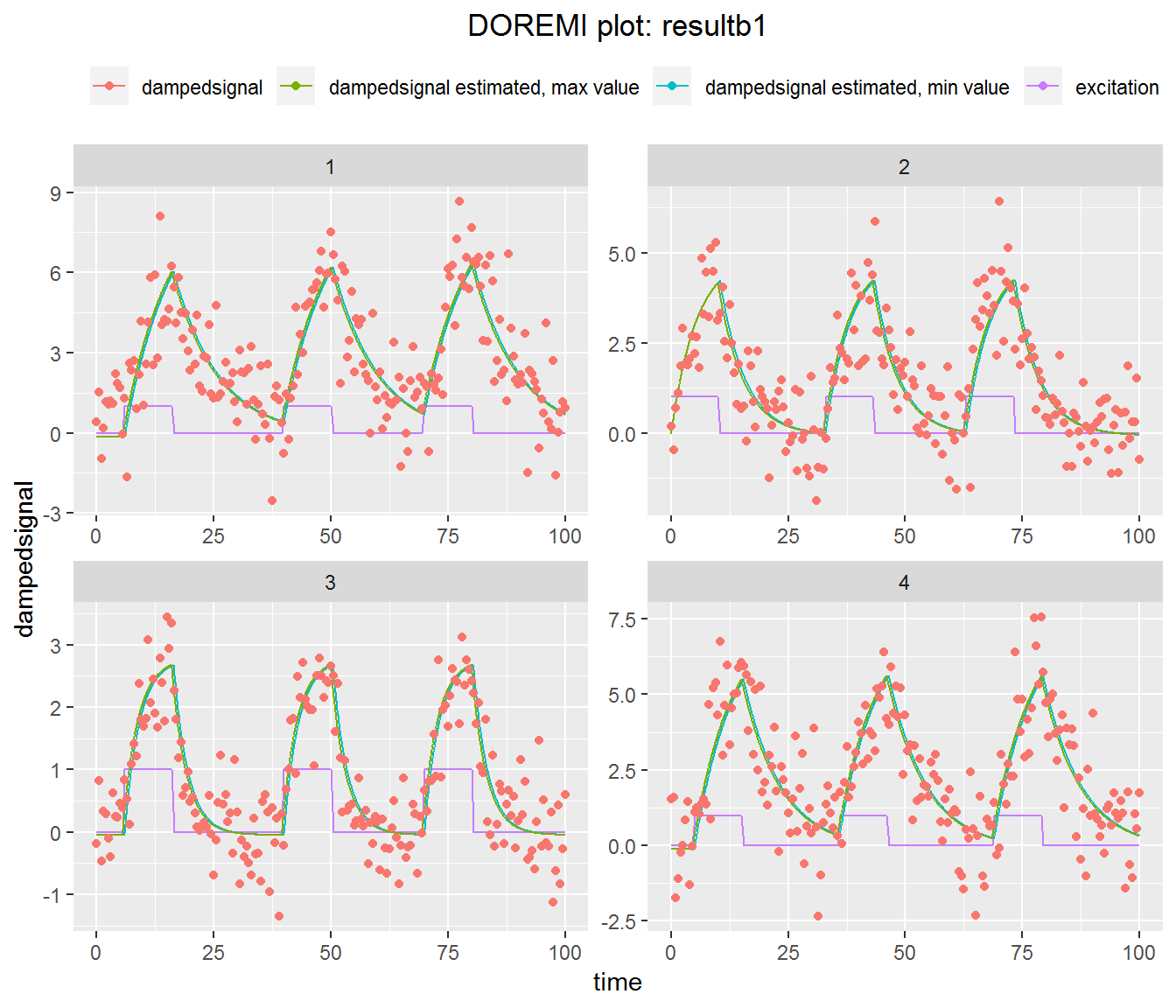The values used to build the estimated signals can be found in resultb1$estimated.

Similarly to the print function, plot applied to a doremi object plots by default the first six individuals contained in the result. If we wish to visualize a single individual or a specific set of individuals, we can specify them by changing the “id” input parameter of the function:

plot(resultb1, id = 3)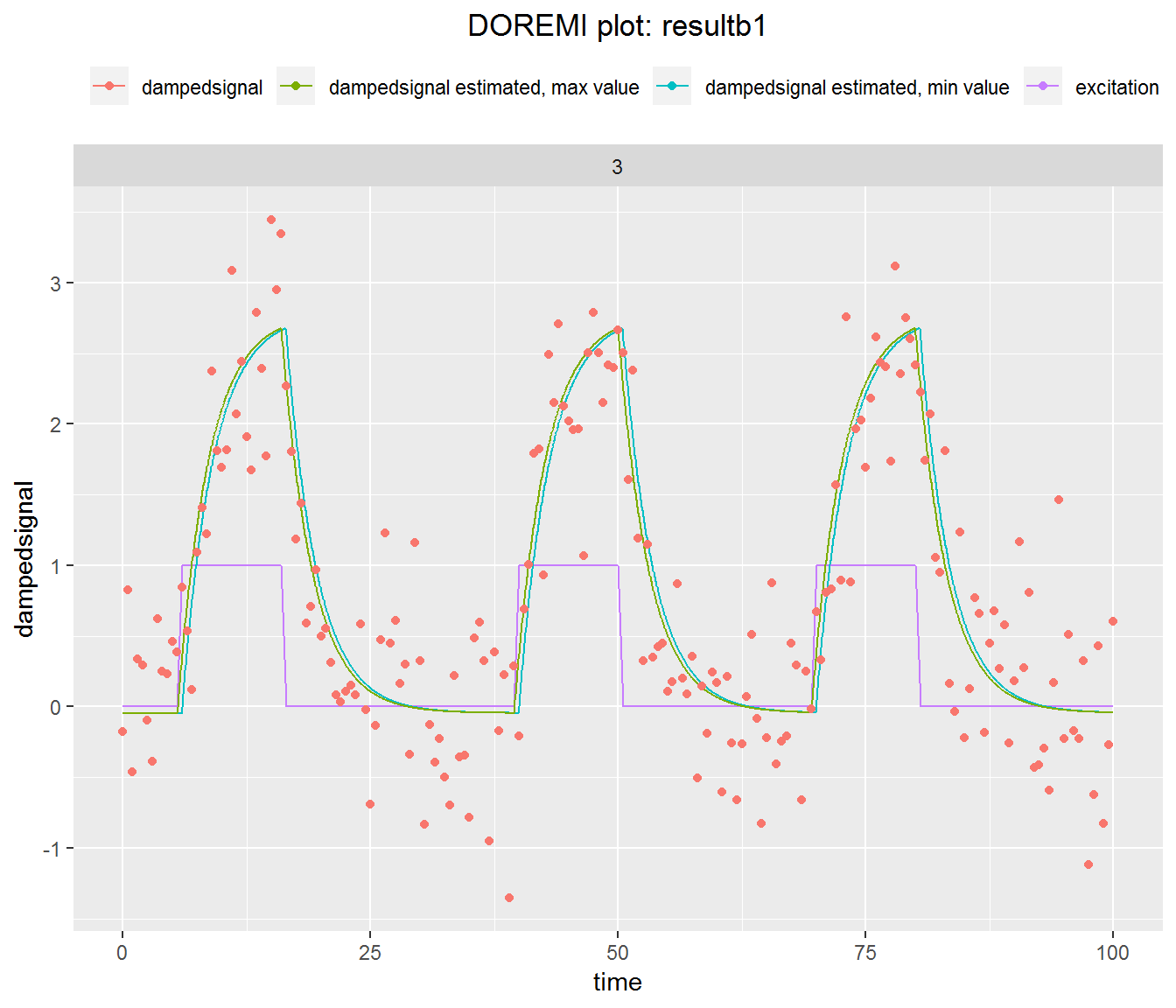plot(resultb1, id = c(1,4))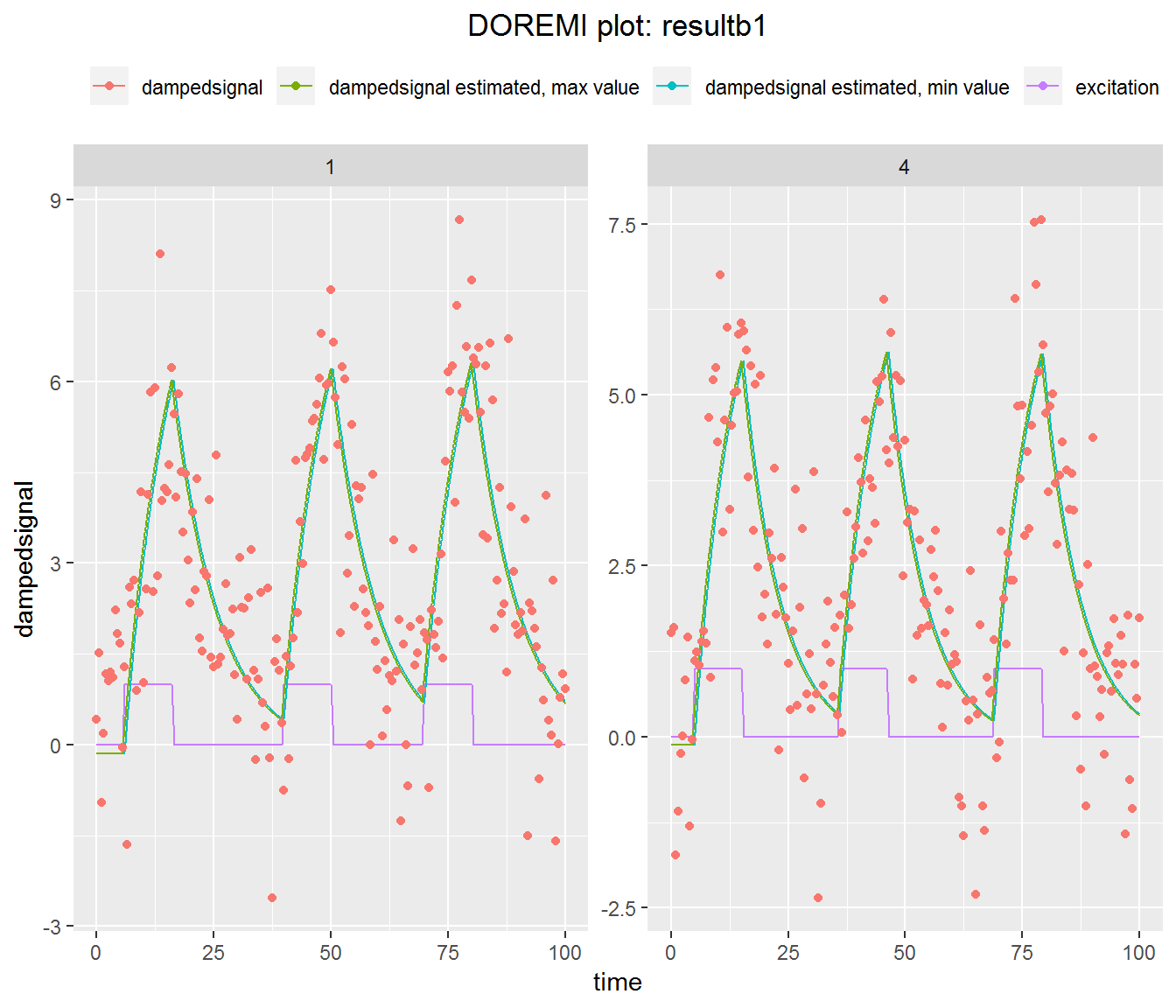## Example 2 - Analyzing data when the signal is subject to several excitations and no noise

In this example, the signal for each individual is set to depend of a linear combination of three excitation signals:

$e(t)=a*e_{1}(t)+b*e_{2}(t)+c*e_{3}(t)$

#Simulating data with these hypothesis
#Generating the three excitation signals:
e1 <- generate.excitation (amplitude = 10,
nexc = 1,
duration = 10,
deltatf = 1,
tmax = 100,
minspacing = 20)
e2 <- generate.excitation (amplitude = 10,
nexc = 1,
duration = 10,
deltatf = 1,
tmax = 100,
minspacing = 20)
e3 <- generate.excitation (amplitude = 10,
nexc = 1,
duration = 10,
deltatf = 1,
tmax = 100,
minspacing = 20)
# Arbitrarily choosing a = 1, b = 2 and c = 5 for the first individual
et1 <- e1$exc + 3 * e2$exc + 5 * e3$exc timt1 <- e3$t  #we can use any of the three time vectors as they are identical for the three excitations
y1 <- generate.remi(dampingtime = 10,
inp